# Solutions of Electricity (Page No- 27) - Physics By Lakhmir Singh, Class 10 Class 10 Notes | EduRev

## Class 10 : Solutions of Electricity (Page No- 27) - Physics By Lakhmir Singh, Class 10 Class 10 Notes | EduRev

The document Solutions of Electricity (Page No- 27) - Physics By Lakhmir Singh, Class 10 Class 10 Notes | EduRev is a part of the Class 10 Course Class 10 Physics Solutions By Lakhmir Singh & Manjit Kaur.
All you need of Class 10 at this link: Class 10

Lakhmir Singh Physics Class 10 Solutions Page No:27

Question 22:
How does the resistance of a wire change when :
(i) its length is tripled ?
(ii) its diameter is tripled ?
(iii) its material is changed to one whose resistivity is three times ?

Solution :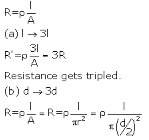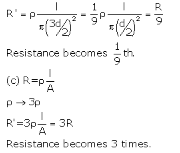Question 23:
Calculate the area of cross-section of a wire if its length is 1.0 m, its resistance is 23 Ω and the resistivity of the material of the wire is 1.84 x 10-6 Ωm.
Solution :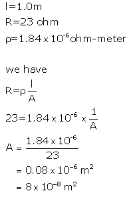Question 24:
(a) Define resistivity. Write an expression for the resistivity of a substance. Give the meaning of each symbol
which occurs in it.
(b) State the SI unit of resistivity.
(c) Distinguish between resistance and resistivity.
(d) Name two factors on which the resistivity of a substance depends and two factors on which it does not depend.
(e) The resistance of a metal wire of length 1 m is 26 Ω at 20°C. If the diameter of the wire is 0.3 mm, what will be the resistivity of the metal at that temperature ?

Solution :

(a) Resistivity,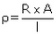where, R is the resistance of the conductor A is the area of cross-section of the conductor I is the length of the conductor.

(b) ohm-meter
1. Resistance is the property of the conductor. while resistivity is the property of the material of the conductor.
2. Resistance of a conductor is the opposition to the flow of electric current through it. Resistivity of a substance is the opposition to the flow of electric current by a rod of that substance which is 1m2  in cross section.
3.Resistance of a conductor depends on length thickness, nature ot material and temperature ot the conductor: while resistvity of substance depends on the nature of the substance and temperature
(d) Resistivity ot a substance depends on the nature of the substance and its temperature. It does not depend on the length or thickness of the conductor.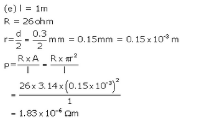Question 33:
A piece of wire of resistance 20 Ω is drawn out so that its length is increased to twice its original length. Calculate the resistance of the wire in the new situation.
Solution :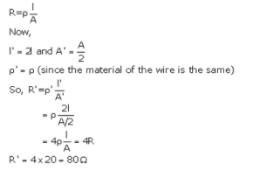Question 34:
The electrical resistivities of three materials P, Q and R are given below :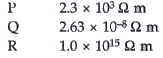Which material will you use for making (a) electric wires (b) handle for soldering iron, and (c) solar cells ? Give reasons for your choices.
Solution :
(a) Material Q with resistivity 2.63 X 10-8 ohm-m can be used for making electric wires because it has very low resistivity.
(b) Material R with resistivity 1.0 X 1015 ohm-m can be used for making handle of soldering iron because it has very high resistivity.
(c) Material P with resistivity 2.3 X 103 ohm-m can be used for making solar cell because it is a semiconductor.

Question 35:
The electrical resistivities of four materials A, B, C and D are given below :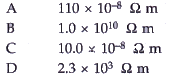Which material is : (a) good conductor (b) resistor (c) insulator, and (d) semiconductor ?
Solution :
(a) Good conductor = C (10 x 10-8ohm-m)
(b) Resistor = A (110 x 10-8 ohm-m)
(c) Insulator= B (1 x 1010 ohm-m)
(d) Semiconductor= D (2.3 x 103 ohm-m)

Question 36:
The electrical resistivities of five substances A, B, C, D and E are given below :
A 5.20 x l0-8 Ω m

Solution :
(a) E is best conductor of electricity due to its least electrical resistivity.
(b) C, because its resistivity is lesser than that of A.
(c) B, because it has the highest electrical resistivity.
(d) C and E, because of their low electrical resistivities.

Offer running on EduRev: Apply code STAYHOME200 to get INR 200 off on our premium plan EduRev Infinity!

94 docs

,

,

,

,

,

,

,

,

,

,

,

,

,

,

,

,

,

,

,

,

,

,

,

,

;